RRB JE EEE (CBT I) Mock Test- 7

# RRB JE EEE (CBT I) Mock Test- 7

Test Description

## 100 Questions MCQ Test RRB JE Mock Test Series Electrical & Electronics Engineering | RRB JE EEE (CBT I) Mock Test- 7

RRB JE EEE (CBT I) Mock Test- 7 for Railways 2022 is part of RRB JE Mock Test Series Electrical & Electronics Engineering preparation. The RRB JE EEE (CBT I) Mock Test- 7 questions and answers have been prepared according to the Railways exam syllabus.The RRB JE EEE (CBT I) Mock Test- 7 MCQs are made for Railways 2022 Exam. Find important definitions, questions, notes, meanings, examples, exercises, MCQs and online tests for RRB JE EEE (CBT I) Mock Test- 7 below.
Solutions of RRB JE EEE (CBT I) Mock Test- 7 questions in English are available as part of our RRB JE Mock Test Series Electrical & Electronics Engineering for Railways & RRB JE EEE (CBT I) Mock Test- 7 solutions in Hindi for RRB JE Mock Test Series Electrical & Electronics Engineering course. Download more important topics, notes, lectures and mock test series for Railways Exam by signing up for free. Attempt RRB JE EEE (CBT I) Mock Test- 7 | 100 questions in 90 minutes | Mock test for Railways preparation | Free important questions MCQ to study RRB JE Mock Test Series Electrical & Electronics Engineering for Railways Exam | Download free PDF with solutions
 1 Crore+ students have signed up on EduRev. Have you?
RRB JE EEE (CBT I) Mock Test- 7 - Question 1

### Ashok remembers his birthday is after 21st December. While his mother remembers his birthday is before 23rd December. On which date of December is his birthday?

Detailed Solution for RRB JE EEE (CBT I) Mock Test- 7 - Question 1 As Ashok remembers, his birthday is after the 21st, and his mother remembers his birthday is before 23rd, therefore, his birthday is on 22nd December.
RRB JE EEE (CBT I) Mock Test- 7 - Question 2

### If in a frequency distribution, the mean and median are 21 and 22 respectively, then its mode is approximately-

Detailed Solution for RRB JE EEE (CBT I) Mock Test- 7 - Question 2 Mode + 2 Mean = 3 Median

Mode = 3 × 22 - 2 × 21 = 66 - 42 = 24.

RRB JE EEE (CBT I) Mock Test- 7 - Question 3

### O is the center of the circle. AC and BD are two chords of the circle intersecting each other at P. If ∠APB=300,∠APB=300, and ∠AOB=450∠AOB=450 then ∠DOC∠DOC is equal to ?

Detailed Solution for RRB JE EEE (CBT I) Mock Test- 7 - Question 3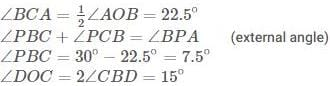RRB JE EEE (CBT I) Mock Test- 7 - Question 4

A does 60% of a work in 45 days. He then calls B, and they together finish the remaining work in 18 days. How long B alone would take to do the whole work?

Detailed Solution for RRB JE EEE (CBT I) Mock Test- 7 - Question 4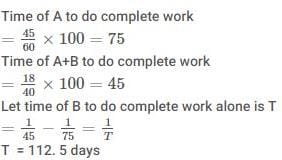RRB JE EEE (CBT I) Mock Test- 7 - Question 5

Abhinash gets 32% marks out of 300 in computer Science. How much marks out of 200 he should get in java language paper so that out of total marks his percentage becomes 46% -

Detailed Solution for RRB JE EEE (CBT I) Mock Test- 7 - Question 5 Abhinash get marks in computer science = 300×32/100=96

Total marks in both subjects = 500×46/100=230

In java = 230−96=134

Required percentage = =134/200×100=67%

RRB JE EEE (CBT I) Mock Test- 7 - Question 6

There are two taps P and Q that can fill a tank in 12 and 24 minutes respectively. Both of them are opened together and after 4 minutes Q is closed. How long more it take for P alone to fill the tank?

Detailed Solution for RRB JE EEE (CBT I) Mock Test- 7 - Question 6 Part of tank filled in 1 minute by tank P = 1/2

Part of the tank filled in 1 minute by tank Q = 1/24

Part of the tank filled by P and Q in 4 minutes =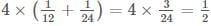Remaining part =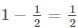Equating Time and part filled, =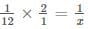x = 6 minutes

RRB JE EEE (CBT I) Mock Test- 7 - Question 7

Find the compound interest on a sum of Rs 425 compounded half yearly for 2 years at the rate of 4%.

Detailed Solution for RRB JE EEE (CBT I) Mock Test- 7 - Question 7 The compound interest for the given condition can be given by,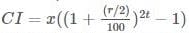Therefore, the required compound interest is,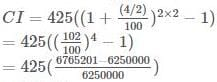= Rs 35.03

Therefore, the compound interest is Rs 35.03.

RRB JE EEE (CBT I) Mock Test- 7 - Question 8

Average age of 6 boys is 14 years. The average age of 11 girls is 12 years. What is the average age (in years) of all boys and girls?

Detailed Solution for RRB JE EEE (CBT I) Mock Test- 7 - Question 8 Average of 6 boys = 14 years

And the average of 11 girls = 12 years.

So, Overall average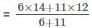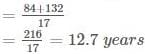RRB JE EEE (CBT I) Mock Test- 7 - Question 9

The value of tan 40. tan 430 .tan 470 .tan 860 is-

Detailed Solution for RRB JE EEE (CBT I) Mock Test- 7 - Question 9

tan4°. tan43°. tan47°. Tan86°

=> tan4°.tan43°. cot4°. cot43° =1

(tan(90° — 9) = cotθ)

RRB JE EEE (CBT I) Mock Test- 7 - Question 10

Profit obtained on selling an article for Rs 310 is equal to the loss incurred on selling that article for Rs 230. What will be the loss percentage when the selling price is Rs 180?

Detailed Solution for RRB JE EEE (CBT I) Mock Test- 7 - Question 10

Let the profit & loss be x

ATQ,

310-x=230+x

2x=80

X=40

CP of article =Rs.270

when the selling price is Rs 180RRB JE EEE (CBT I) Mock Test- 7 - Question 11

Diameter of the cylindrical vessel is 14 cm. having some water. When a sphere of iron is totally submerged in this water level of water increased by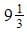cm. What is the radii of the sphere?

Detailed Solution for RRB JE EEE (CBT I) Mock Test- 7 - Question 11

d = 14 then r= 7

let the radius of sphere = R

∴ the volume of sphere = volume of cylinder

∴ volume of sphere = volue of cylinder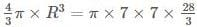R3 = 343

R = 7 cm.

RRB JE EEE (CBT I) Mock Test- 7 - Question 12

The given figure, AB = 16 cm, AC = 12 cm, BC = 14 cm, then the length of median AD will be -

Detailed Solution for RRB JE EEE (CBT I) Mock Test- 7 - Question 12

(16)2 + (16)2 = 2(AD2 + CD2)

256 + 144 = 2AD2 + 98

RRB JE EEE (CBT I) Mock Test- 7 - Question 13

Directions

Each question is followed by 2 statements. Go through these questions and answer the questions as per the instructions : choose your answer as: (A) If the question can be answered by using only statement (I), but not by using statement (II) alone. (B) If the question can be answered by using only statement (II), but not by using statement (I) alone. (C) If the question can be answered by using both the statements together, but cannot be answered by using either statement alone. (D) If the question cannot be answered by using both the statements together.

What is the number?

A. The product of two digits of a number is 27.

B. The sum of the two digits is 12. The ratio of the ten's digit to the unit digit is 3:1.

Detailed Solution for RRB JE EEE (CBT I) Mock Test- 7 - Question 13

Let the ten's and unit’s digit be x and y respectively.

From A, xy = 27

From B, x + y = 12 and Now,

3y + y=12

4y=12 =>y=3

x=3y=9

Number = 93

Thus, B alone gives the answer and A alone does not give the answer

RRB JE EEE (CBT I) Mock Test- 7 - Question 14

Two places R and S are 800 km apart from each other. Two persons start from R towards S at an interval of 2 hours. Whereas A leaves R for S before B. The speeds of A and B are 40 km/h and 60 km/h respectively. B overtakes A at M, which is on the way from R to S. What is the distance from R, where B overtakes A?

Detailed Solution for RRB JE EEE (CBT I) Mock Test- 7 - Question 14 speed of A=40 km/hr

speed of B= 60 km/hr

In two hour A cover distance= 2 x 40 = 80km

relative speed of A and B=60-40=20 km/hr

the A and B meet at point M in hours= 80/20 = 4

In 4 hours B cover distance= 4 x 60 = 240 km

RRB JE EEE (CBT I) Mock Test- 7 - Question 15

The area of a square is 72.25 cm2 . Find its perimeter (in cm).

Detailed Solution for RRB JE EEE (CBT I) Mock Test- 7 - Question 15 Area of square = side side =72.25

Side =8.5

Perimeter = 4 side = 4 8.5=34cm

RRB JE EEE (CBT I) Mock Test- 7 - Question 16

Arrange the fractions 3/4, 5/12, 13/16, 16/29, 3/8 in their ascending order of magnitude.

Detailed Solution for RRB JE EEE (CBT I) Mock Test- 7 - Question 16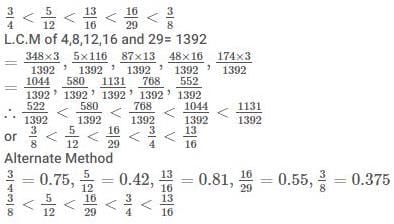RRB JE EEE (CBT I) Mock Test- 7 - Question 17

The concentrations of acid in two solution A and B is 19 percent and 9 percent respectively.In what ratio solution A and B should be mixed to get a solution having 17 percent acid?

Detailed Solution for RRB JE EEE (CBT I) Mock Test- 7 - Question 17 Using Allegation method-

The required ratio=8:2=4:1

RRB JE EEE (CBT I) Mock Test- 7 - Question 18

Find the greatest number which on dividing 1580 and 3800 leaves remainders 8 and 1 respectively

Detailed Solution for RRB JE EEE (CBT I) Mock Test- 7 - Question 18 HCF of (1580-8) and (3800-1) will be the greatest number

So HCF of 1572 and 3799

So, 131 Is the number.

RRB JE EEE (CBT I) Mock Test- 7 - Question 19

What must be added to each term of the ratio 2 : 5 so that it may equal to 5 : 6 ?

Detailed Solution for RRB JE EEE (CBT I) Mock Test- 7 - Question 19 Suppose x must be added.

Then, 2+x/5+x=5/6

12 + 6x = 25 + 5x

x = 13

RRB JE EEE (CBT I) Mock Test- 7 - Question 20

The angle of elevation of the top of a tower at a distance of 25 m from its foot is 600 . The approximate height of the tower is -

Detailed Solution for RRB JE EEE (CBT I) Mock Test- 7 - Question 20 We Know that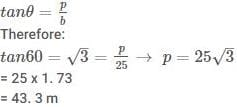RRB JE EEE (CBT I) Mock Test- 7 - Question 21

How many whole numbers are there between 52 and 356 which are exactly divisible by 6?

Detailed Solution for RRB JE EEE (CBT I) Mock Test- 7 - Question 21 First whole number which is divisible by 6 between 52 and 356 is 54

Last whole number which is divisible by 6 between 52 and 356 is 354

So, by using AP formula:-

Last term = first term + (number of terms-1) × Common difference

354 = 54 + (n-1) × 6

300 = (n-1) × 6

50= (n-1)

So, n =51

Therefore,

There are 51 whole numbers between 52 and 356 which are exactly divisible by 6.

RRB JE EEE (CBT I) Mock Test- 7 - Question 22

Directions

In the following problems, there is one question and some statements given below the question. You have to decide whether the data given in the statements is sufficient to answer the question. Read all the statements carefully and find out which of the statements is/are sufficient to answer the given question. Choose the correct alternative for each question.

Question:

What will be the total weight of 10 poles, each of the same weight ?

Statements:

I. One-fourth of the weight of each pole is 5 kg.

II. The total weight of three poles is 20 kilograms more than the total weight of two poles.

Detailed Solution for RRB JE EEE (CBT I) Mock Test- 7 - Question 22 From I, we conclude that weight of each pole = (4 x 5) kg = 20 kg.

So, total weight of 10 poles = (20 x 10) kg = 200 kg.

From II, we conclude that:

Weight of each pole = (weight of 3 poles) - (weight of 2 poles) = 20 kg.

So, total weight of 10 poles = (20 x 10) kg = 200 kg.

RRB JE EEE (CBT I) Mock Test- 7 - Question 23

B starts some business by investing Rs 90000. After 4 months, D joins business by investing Rs 80000. At the end of the year, in what ratio will they share the profit?

Detailed Solution for RRB JE EEE (CBT I) Mock Test- 7 - Question 23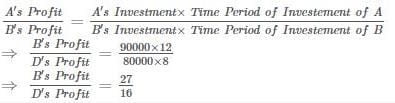RRB JE EEE (CBT I) Mock Test- 7 - Question 24

Calculate the total numbers of prime factors in the expression (9)11 × (5)7 × (3)2 × (17)2

Detailed Solution for RRB JE EEE (CBT I) Mock Test- 7 - Question 24 (9)11 x (5)7 x (7)5 x (3)2 x (17)2

⇒ (3)22 x (5)7 x (7)5 x (3)2 x (17)2

Total no. of prime factors, n = Sum of powers

∴ n = 22+7 + 5 + 2 + 2

∴ n = 38

RRB JE EEE (CBT I) Mock Test- 7 - Question 25

What is the net discount (in %) for successive discounts of 30% and 50%?

Detailed Solution for RRB JE EEE (CBT I) Mock Test- 7 - Question 25 Cumulated Discount1 + Discount2 -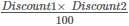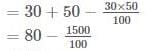= 80 - 15

= 65%

RRB JE EEE (CBT I) Mock Test- 7 - Question 26

In a mixture of 45 litres, the ratio of liquid A and liquid B is 7 : 2. If 11 litres of liquid B is added to the mixture, then what will be the ratio of liquid A and liquid B in the new mixture?

Detailed Solution for RRB JE EEE (CBT I) Mock Test- 7 - Question 26 Let Liquid A and Liquid B be 7x and 2x respectively.

ATQ,

7x+2x = 45

9x = 45

So, x = 5

And, Liquid A is 35 and Liquid B is 10 litres respectively.

Liquid B becomes 21 litres.

Therefore,

Liquid A/Liquid B = 35/21 = 5/3

RRB JE EEE (CBT I) Mock Test- 7 - Question 27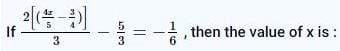Detailed Solution for RRB JE EEE (CBT I) Mock Test- 7 - Question 27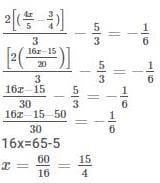RRB JE EEE (CBT I) Mock Test- 7 - Question 28

A shopkeeper offer Rs. 19% discount on a mobile phone with a marked price of Rs. 7400. If he still earns a profit of Rs. 500, what is the cost price of the phone?

Detailed Solution for RRB JE EEE (CBT I) Mock Test- 7 - Question 28 Selling Price = Marked Price × (100-Discount) %

Therefore,

SP = 7400 × (100-19) %

SP=7400×81/100

SP = 5994

So,

Cost Price = Selling Price - Profit

CP = 5994-600

CP = 5494

RRB JE EEE (CBT I) Mock Test- 7 - Question 29

The following equation is incorrect. Which two signs should be interchanged to correct the equation?

12 + 8 - 25 ÷ 10 x 18 = 14

Detailed Solution for RRB JE EEE (CBT I) Mock Test- 7 - Question 29 12 + 8 - 25 ÷ 10 x 18

After interchanging signs,

12 + 8 x 25 ÷ 10-18

⇒ 12 + 8 x 25/10 - 18

⇒ 12 + 20 18

⇒ 14

RRB JE EEE (CBT I) Mock Test- 7 - Question 30

Two pipes can fill a tank in 1hr. and 72 min respectively both the pipes are open together but due to some dust in pipe, the efficiency pipes are decreased to 5/6 and 9/10 of their actually efficiency of respectively. After some time the dust in both pipes get clear together and both pipes run with their full efficiency and then reaming the tank can be filled in 31 min. Find in how much time it takes to clear dust.

Detailed Solution for RRB JE EEE (CBT I) Mock Test- 7 - Question 30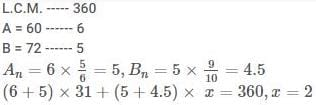RRB JE EEE (CBT I) Mock Test- 7 - Question 31

In the following question, select the related numbers from the given alternatives.

5327 : 2105 ∷ 7134 : ?

Detailed Solution for RRB JE EEE (CBT I) Mock Test- 7 - Question 31

5*3*2*7= 210

And, 3+2=5

Written as → 2105

Similarly,

7*1*3*4= 84

3+1= 4

Written as → 844

RRB JE EEE (CBT I) Mock Test- 7 - Question 32

Which of the words in the following options can be formed using the given word ?

CORRESPONDING

Detailed Solution for RRB JE EEE (CBT I) Mock Test- 7 - Question 32 There is no ‘T’ in the given word. So, CORRECT and REPENT cannot be formed.

There is only one ‘S’ in the given word. So, RESPONSE cannot be formed.

RRB JE EEE (CBT I) Mock Test- 7 - Question 33

The following figure consists of a 3x3 matrix. The matrix consists of 9 different figures. The figures are such that 3 of them bear a similar relation and form group 1, another 3 figures bear a relation and form group 2 and similarly the rest 3 bear another relationship and form group 3. Find the option which shows all three groups in the correct sequence.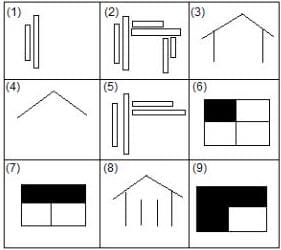Detailed Solution for RRB JE EEE (CBT I) Mock Test- 7 - Question 33

Group 1 - (8, 3, 4) - one by one in each step, lines are removed.

Group 2 - (6, 7, 9) - one by one in each step, each block is shaded.

Group 3 - (2, 5, 1) - one by one in each step, the parallel blocks are removed.

RRB JE EEE (CBT I) Mock Test- 7 - Question 34

In the following question, select the related letter/word/number from the given alternatives.

Hooghly : Jute :: Ahmedabad : ?

Detailed Solution for RRB JE EEE (CBT I) Mock Test- 7 - Question 34 Hoogly is related to jute productions, similarly Ahmedabad is related to cotton type if productions.
RRB JE EEE (CBT I) Mock Test- 7 - Question 35

If in a certain code 'MEDIUM' is coded as '632516' and 'HOTEL' is coded as '94738' then how will 'LIMITED' be coded as in the same code?

Detailed Solution for RRB JE EEE (CBT I) Mock Test- 7 - Question 35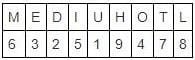The code for LIMITED will be '8565732'

RRB JE EEE (CBT I) Mock Test- 7 - Question 36

DIRECTIONS: Study the following information to answer the given questions

In a certain code:

‘swords hidden in area’ is written as ‘white black yellow red’

‘ready swords for attack’ is written as ‘grey pink red green’

‘hidden for own safety’ is written as ‘silver grey violet white’

‘own area under attack’ is written as ‘violet blue pink black’

‘black pink yellow’ could be a code for which of the following?

Detailed Solution for RRB JE EEE (CBT I) Mock Test- 7 - Question 36

From 1 & 2

swords = red

From 1 & 3

hidden = white

From 3 & 4

own = violet

From 1 & 4

area = black

From 1

in= yellow

From 2 & 3

for= grey

From 2

From 3

safety = silver

From 4

under = blue

So, ‘black pink yellow’ is ‘Area in attack’

RRB JE EEE (CBT I) Mock Test- 7 - Question 37

How many Sundays are there in between 3rd June 2016 and 3rd October 2016, if it is given that 1st June 2016 was Wednesday.

Detailed Solution for RRB JE EEE (CBT I) Mock Test- 7 - Question 37 1st June 2016 = Wednesday

3rd June 2016 = Friday

Number of days from 3rd June 2016 and 3rd October 2016 = 30+31+31+30= 122

122 when divided by 7, we get, remainder = 122 - 119 = 3

And, 119/7 = 17

Thus, there will be 17 full weeks in between which means 17 Sunday.

But, 3 remainder is there, which gives one more Sunday, as Friday + 3 = Monday (in between there is Sunday)

Total Sundays = 17+1= 18

RRB JE EEE (CBT I) Mock Test- 7 - Question 38

In each of the questions below, one statement is given, followed by two conclusions which may or may not follow. From the options below, choose the one that reflects the correct choice of conclusion(s) that follows/follow.

P is a company which sells mattresses. In its ads, it says it is making dreams.

I. Taglines and slogans have always been an integral part of companies' marketing communication.

II. The hype of sloganeering bears no resemblance to the reality of actual business.

Detailed Solution for RRB JE EEE (CBT I) Mock Test- 7 - Question 38 I- it cannot be concluded as the main statement talks of just one company whereas statement I is a general statement encompassing all companies. II- Whether the tagline is a hype or not is out of scope of the conclusion. Hence, (d) is the right answer.
RRB JE EEE (CBT I) Mock Test- 7 - Question 39

In each of the questions given below, there are two statements followed by an inference. Mark the correct option accordingly.

(a) Managers are encouraged to develop and maintain good working relationships with employees.

(b) Good working relationships result in stronger commitment and support, as well as better performance.

Inference: A company which has good working relationships between managers and employees performs better than the one which doesn't have them.

Detailed Solution for RRB JE EEE (CBT I) Mock Test- 7 - Question 39 Since it is mentioned in statement (b) that good working relationships result in better performance; hence, we can infer that statement mentioned in 'Inference'. Therefore, (a) is the right answer.
RRB JE EEE (CBT I) Mock Test- 7 - Question 40

In the following question, select the related letters from the given alternatives.

FISJ : DFVH ∷ RWEM : ?

Detailed Solution for RRB JE EEE (CBT I) Mock Test- 7 - Question 40

F - 2=D

I - 3=F

S + 3=E

J - 2=H

Similarly,

R - 2=P

W - 3=T

E + 3=H

M - 2=K

RRB JE EEE (CBT I) Mock Test- 7 - Question 41

Each of the following questions consists of a question and two statements numbered I and II. You have to tell whether the data provided in the statements is sufficient to answer the question or not.

Five shapes - pentagon, triangle, square, rectangle and circle, are drawn on a paper. Each shape has different size.

Which shape is the smallest?

I. Circle is larger than Triangle, which is smaller than both square and rectangle. Pentagon is smaller than triangle.

II. Neither square nor circle, is the largest. Pentagon is smaller than circle, which is smaller than square.

Detailed Solution for RRB JE EEE (CBT I) Mock Test- 7 - Question 41

From I alone, we get -

→ Circle is larger than Triangle, which is smaller than both square and rectangle.

Circle, square, rectangle > Triangle

Also,

→ Pentagon is smaller than triangle.

So, we get that Pentagon is the smallest.

Circle, square, rectangle > Triangle > Pentagon

Hence, I alone is sufficient.

From II alone, we get -

→ Pentagon is smaller than circle, which is smaller than square.

Square > Circle > Pentagon

→ Neither square nor circle, is the largest.

Thus, either rectangle or triangle is the largest.

And, the smallest can be either Pentagon or triangle or rectangle.

Thus, II alone is not sufficient.

RRB JE EEE (CBT I) Mock Test- 7 - Question 42

Suraj walked 3km towards west and reached point A and he turned right and walked another 5km to reach point B. From point B he took a left turn and walked another 7km to point C. After covering another 4km he rested for a while. How much distance had Suraj covered from point A till the time he stopped?

Detailed Solution for RRB JE EEE (CBT I) Mock Test- 7 - Question 42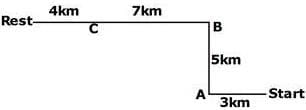Required distance = 5+7+4 = 16km

RRB JE EEE (CBT I) Mock Test- 7 - Question 43

In each of the questions below are given some statements followed by some conclusions. You have to take the given statements to be true even if they seem to be at variance from commonly known facts. Read all the conclusions and then decide which of the given conclusions logically follows from the given statements disregarding commonly known facts.

Statements:

All lights are bulbs.

No bulb is a lamp.

All lamps are torches.

Conclusions:

I. No torch is a bulb.

II. No light is a lamp.

Detailed Solution for RRB JE EEE (CBT I) Mock Test- 7 - Question 43 A possible representation is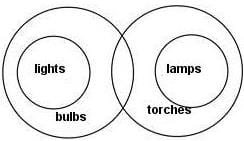If some lights are lamps, some lamps would be bulbs, which contradicts the given statement.

RRB JE EEE (CBT I) Mock Test- 7 - Question 44

The numbers in the matrix given below follow a certain trend row-wise and/or column-wise. Study the trend and choose the number which will replace the question mark.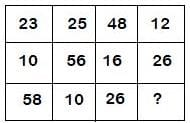Detailed Solution for RRB JE EEE (CBT I) Mock Test- 7 - Question 44 23+25+48+12 = 108

10+56+16+26 = 108

Similarly,

58+10+26+ (?) = 108

We get, (?)= 108 - 94 = 14

RRB JE EEE (CBT I) Mock Test- 7 - Question 45

If 'x' means 'addition', '-' means 'division', '/' means 'subtraction' and '+' means 'multiplication', then what will be the value of the expression '6+2/12-24+4x3'?

Detailed Solution for RRB JE EEE (CBT I) Mock Test- 7 - Question 45 6+2/12-24+4x3= 6x2-12/24x4+3= 6x2-2+3= 12-2+3= 15-2=13
RRB JE EEE (CBT I) Mock Test- 7 - Question 46

In a certain code language, "Felicity Smoke Malcolm Merlyn" is coded as "Aa Cc Ff Rr", "Oliver Queen Malcolm Smoke" is coded as "Dd Gg Ff Aa" and "Arrow Sara Queen Malcolm" is coded as "Ww Zz Gg Ff". Find what will be the code for "Smoke Queen"?

Detailed Solution for RRB JE EEE (CBT I) Mock Test- 7 - Question 46

Given;

"Felicity Smoke Malcolm Merlyn" is coded as "Aa Cc Ff Rr" (I)

"Oliver Queen Malcolm Smoke" is coded as "Dd Gg Ff Aa" (II)

"Arrow Sara Queen Malcolm" is coded as "Ww Zz"Gg Ff" .. (III)

From (I), (II) and (III);

Malcolm = Ff

Now,

From (I) and (II);

Smoke = Aa

From (II) and (III);

Queen = Gg

RRB JE EEE (CBT I) Mock Test- 7 - Question 47

Identify the diagram that best represents the relationship among the given classes.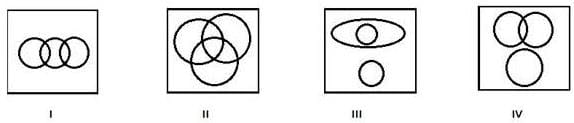Detailed Solution for RRB JE EEE (CBT I) Mock Test- 7 - Question 47 Some children are students while some adults are also students.

We get,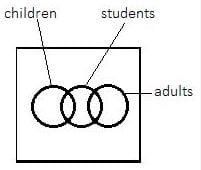RRB JE EEE (CBT I) Mock Test- 7 - Question 48

Each question given below consists of a statement, followed by two arguments numbered I and II. Decide which of the arguments is a 'strong' argument and which is a 'weak' argument and choose the corresponding option as your answer.

Should loud music be permitted at weddings?

I. No, because it causes noise pollution which may affect other people in the neighbourhood.

II. Yes, because weddings cannot be enjoyed without loud music.

Detailed Solution for RRB JE EEE (CBT I) Mock Test- 7 - Question 48 I is a strong argument as it gives a valid reason why it would be wrong to permit loud music at weddings. II is a weak argument as it presupposes that loud music is imperative in order to enjoy at a wedding, which has no basis. A is the right answer.
RRB JE EEE (CBT I) Mock Test- 7 - Question 49

How many triangles are there in the given figure?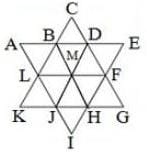Detailed Solution for RRB JE EEE (CBT I) Mock Test- 7 - Question 49

∆ABL, ∆BCD, ∆DEF, ∆FGH, ∆HIJ, ∆JKL, ∆KCG, ∆AEI, ∆LBM, ∆BMD,

∆DMF, ∆MFH, ∆HJM, ∆JLM, ∆ADJ, ∆BEH, ∆LFI, ∆CLF, ∆KBH, ∆JDG

RRB JE EEE (CBT I) Mock Test- 7 - Question 50

While introducing a man to Geeta, Mohan said "He is the only son of the paternal grandfather of the daughter of sister of my mother." How is that man related to Mohan?

Detailed Solution for RRB JE EEE (CBT I) Mock Test- 7 - Question 50 That person is the husband of Mohan's mother's sister so he is the maternal uncle of Mohan.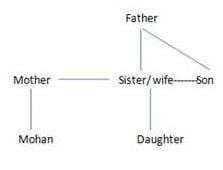RRB JE EEE (CBT I) Mock Test- 7 - Question 51

There are two sets of figures namely problem Figure containing five figures 1, 2, 3, 4, 5 and answer figures (a), (b), (c), (d). You have to select one Figure from the answer set which will continue the same series as given in problem set figures.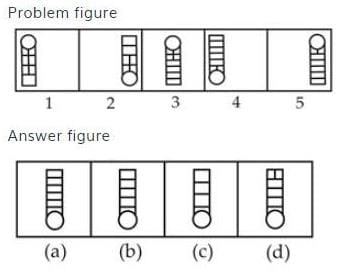Detailed Solution for RRB JE EEE (CBT I) Mock Test- 7 - Question 51 Problem figure 2 is reverse of figure 1 with same number of horizontal lines and vertical linesdecreases by one row. Similarly, Figure 4 is revers of figure 3 and symmetrical changes in pattern of lines. Therefore, answer figure (a) is reverse of problem figure 5.
RRB JE EEE (CBT I) Mock Test- 7 - Question 52

Each of the following questions consists of a question and two statements numbered I and II. You have to tell whether the data provided in the statements is sufficient to answer the question or not.

How is 'salty bitter' coded in the given code language?

I. 'sugar is sweet' is coded as '76 12 98'; and, 'food is salty' is coded as '98 54 15'.

II. 'salty junk food' is coded as '34 15 54'; and, 'bitter junk food' is coded as '54 19 34'.

Detailed Solution for RRB JE EEE (CBT I) Mock Test- 7 - Question 52 From I alone, we get,

'sugar is sweet' is coded as '76 12 98'

'food is salty' is coded as '98 54 15'.

We get,

Is= 98

We can't get the answer from I alone.

From II alone -

'bitter junk food' is coded as '54 19 34'

'salty junk food' is coded as '34 15 54'

Junk /food = 34/54

Salty = 15

Bitter = 19

Hence, II alone is sufficient.

RRB JE EEE (CBT I) Mock Test- 7 - Question 53

In the following question, four figures marked A, B, C and D, are given. Among these, three figures follow the same pattern and remaining one figure doesn't follow that pattern and hence, is odd. Mark the option which denotes the odd figure.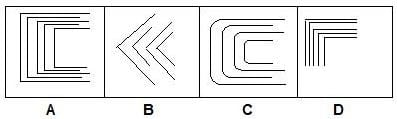Detailed Solution for RRB JE EEE (CBT I) Mock Test- 7 - Question 53 The size of lines increases and decreases alternately in all, except in D.
RRB JE EEE (CBT I) Mock Test- 7 - Question 54

The series is given, with one term missing. Choose amongst the given responses and complete the series.

ABBD, BCDF, CDFH, DEHJ, __.

Detailed Solution for RRB JE EEE (CBT I) Mock Test- 7 - Question 54

In 1st term;

1st and 2nd letters are: -

A = 1

B = 2

3rd and 4th letters are: -

1 x 2 = 2 = B

2 x 2 = 4 = D

In 2nd term;

1st and 2nd letters are: -

B = 2

C = 3

3rd and 4th letters are: -

2 x 2 = 4 = D

3 x 2 = 6 = F

In 3rd term;

1st and 2nd letters are: -

C = 3

D = 4

3rd and 4th letters are: -

3 x 2 = 6 = F

4 x 2 = 8 = H

In 4th term;

1st and 2nd letters are: -

D = 4

E = 5

3rd and 4th letters are: -

4 x 2 = 8 = H

5 x 2 = 10 = J

In 5th term;

1st and 2nd letters are: -

E = 5

F = 6

3rd and 4th letters are: -

5 x 2 = 10 = J

6 x 2 = 12 = L

So, we get: - EFJL

RRB JE EEE (CBT I) Mock Test- 7 - Question 55

Complete the following series:

Y, F, T, G, O, H, J, I, ?, ?

Detailed Solution for RRB JE EEE (CBT I) Mock Test- 7 - Question 55 Y _ _ _ _ T _ _ _ _ O _ _ _ _ J _ _ _ _ E

And,

F G H I J

We get, answer - E, J

RRB JE EEE (CBT I) Mock Test- 7 - Question 56

The first Viceroy of India was.

Detailed Solution for RRB JE EEE (CBT I) Mock Test- 7 - Question 56 The first viceroy of India was lord Cannings. After the mutiny of 1857, the title of viceroy was created in 1858. Before 1858, it was East India Company who was ruling large parts of India and the head of administration of the East India Company was called Governor general. This office was created in 1773 (by regulating act)
RRB JE EEE (CBT I) Mock Test- 7 - Question 57

15th edition of Pravasi conference will be held in:

Detailed Solution for RRB JE EEE (CBT I) Mock Test- 7 - Question 57 15th edition of Pravasi conference will be held in Varanasi close to the site of ‘Ardh Kumbh’.
RRB JE EEE (CBT I) Mock Test- 7 - Question 58

How many members of the Anglo-Indian community can be nominated by the President of India to the Parliament?

Detailed Solution for RRB JE EEE (CBT I) Mock Test- 7 - Question 58 The Constitution provides that the maximum strength of the House be 552 members. up to 525 members represent of the territorial constituencies in States, up 20 members represent the Union Territories and no more than two members from Anglo-Indian community can be nominated by the President of Indian if he or she feels that the community is not adequately represented.
RRB JE EEE (CBT I) Mock Test- 7 - Question 59

Which Amendment of the Constitution deals with Political defections?

Detailed Solution for RRB JE EEE (CBT I) Mock Test- 7 - Question 59 The fifty-second Amendment 1985, inserted the Tenth Schedule in the constitution regarding provisions as to disqualification on the grounds of defection.
RRB JE EEE (CBT I) Mock Test- 7 - Question 60

Which is the highest peak of India?

Detailed Solution for RRB JE EEE (CBT I) Mock Test- 7 - Question 60 Kangchenjunga is the highest peak of India.
RRB JE EEE (CBT I) Mock Test- 7 - Question 61

Where will be the two new Indian Institutes of Science Education & Research (IISERs) established?

Detailed Solution for RRB JE EEE (CBT I) Mock Test- 7 - Question 61 The Union Cabinet chaired by the Prime Minister Shri Narendra Modi has approved establishment and operationalistion of permanent campuses of the two new Indian Institutes of Science Education & Research (IISERs) at Tirupati (Andhra Pradesh) and Berhampur (Odisha).
RRB JE EEE (CBT I) Mock Test- 7 - Question 62

The older alluvium is called

Detailed Solution for RRB JE EEE (CBT I) Mock Test- 7 - Question 62 The older alluvium is called the Bhangar
RRB JE EEE (CBT I) Mock Test- 7 - Question 63

In Shivaji’s Council of ministers the Prime minister was called.

Detailed Solution for RRB JE EEE (CBT I) Mock Test- 7 - Question 63 The Ashta Pradhan was a council of minisless that administered the Maratha empire.
RRB JE EEE (CBT I) Mock Test- 7 - Question 64

Which river of India is called Dakshin Ganga?

Detailed Solution for RRB JE EEE (CBT I) Mock Test- 7 - Question 64 Godavari is considered the Dhakshin (Southern) Ganga and it is the largest river of the peninsular India. It rises near Nasik in Maharashtra.
RRB JE EEE (CBT I) Mock Test- 7 - Question 65

Money Bills are described in the Article _________.

Detailed Solution for RRB JE EEE (CBT I) Mock Test- 7 - Question 65 Money Bills are described in the Article 110
RRB JE EEE (CBT I) Mock Test- 7 - Question 66

Who was the First Indian Nobel Prize winner?

Detailed Solution for RRB JE EEE (CBT I) Mock Test- 7 - Question 66 Rabindranath Tagore (1861-1941) was a poet, philosopher, educationist, artist and social activist. In 1913, he was awarded the Nobel Prize in Literature. He was the first person of non-Western heritage to be awarded a Nobel Prize.
RRB JE EEE (CBT I) Mock Test- 7 - Question 67

Iltutmish established a centre of learning at:

Detailed Solution for RRB JE EEE (CBT I) Mock Test- 7 - Question 67 Iltutmish established a centre of learning at Patna.
RRB JE EEE (CBT I) Mock Test- 7 - Question 68

The Tapti river rises from the—

Detailed Solution for RRB JE EEE (CBT I) Mock Test- 7 - Question 68

The Tapti River is one of the major rivers of peninsular India with a length of around 724 kilometres (450 mi). It is one of only three rivers in peninsular India that run from east to west - the others being the Narmada River and the Mahi River. The river rises in the eastern Satpura Range of southern Madhya Pradesh state, and flows westward, draining Madhya Pradesh's Nimar region, Maharashtra's Kandesh and east Vidarbha regions in the northwest corner of the Deccan Plateau and south Gujarat, before emptying into the Gulf of Cambay of the Arabian Sea, in the Surat District of Gujarat. The river, along with the northern parallel Narmada River, form the boundaries between North and South India. The Western Ghats or Sahyadri range starts south of the Tapti River near the border of Gujarat and Maharashtra. The Tapti (Tapi) River empties into the Gulf of Khambhat near the city of Surat in Gujarat.

RRB JE EEE (CBT I) Mock Test- 7 - Question 69

World Tsunami Awareness Day being observed on __________.

Detailed Solution for RRB JE EEE (CBT I) Mock Test- 7 - Question 69 World Tsunami Awareness Day is being observed today across the world, including Pakistan, aiming at minimizing the number of people affected by disasters worldwide.
RRB JE EEE (CBT I) Mock Test- 7 - Question 70

Where was 46th “Know India Programme” in March, 2018 held?

Detailed Solution for RRB JE EEE (CBT I) Mock Test- 7 - Question 70 The 46th “Know India Programme” in March, 2018 was held in New Delhi.
RRB JE EEE (CBT I) Mock Test- 7 - Question 71

In case of spherical mirrors, focal length is _______ of its radius of curvature image.

Detailed Solution for RRB JE EEE (CBT I) Mock Test- 7 - Question 71 In case of spherical mirrors, focal length is half its radius of curvature image. Radius curvature of a mirror is defined as the radius of that sphere of which the mirror forms a part.
RRB JE EEE (CBT I) Mock Test- 7 - Question 72

Lemon contains Citric acid in the same way Vinegar contain ___________.

Detailed Solution for RRB JE EEE (CBT I) Mock Test- 7 - Question 72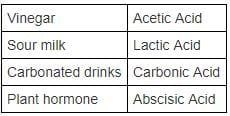RRB JE EEE (CBT I) Mock Test- 7 - Question 73

When a pendulum is at either side of the peak in its oscillation, its potential energy is _______ while kinetic energy is _______.

Detailed Solution for RRB JE EEE (CBT I) Mock Test- 7 - Question 73 During the oscillatory motion of a pendulum, energy balance is as follows-

The peak position: its potential energy is maximum while kinetic energy is minimum

The middle rest position: its potential energy is minimum while kinetic energy is maximum

RRB JE EEE (CBT I) Mock Test- 7 - Question 74

________________ are a species of plant which are adapted to life in a dry or physiologically dry habitat.

Detailed Solution for RRB JE EEE (CBT I) Mock Test- 7 - Question 74 Xerophytes are the plant species adapted to life in a dry or physiologically dry habitat like salt marsh, saline soil desert or ice-covered areas by means of mechanisms to prevent water loss or to store available water.

Examples Cacti, Pineapple, Pine trees etc

RRB JE EEE (CBT I) Mock Test- 7 - Question 75

Which of the following are examples of polymers?

Detailed Solution for RRB JE EEE (CBT I) Mock Test- 7 - Question 75 Polymer is any useful chemical made of many repeating units- either 1-dimensional, 2-dimensional or 3-dimensional called monomers.

Nylon, Polyvinyl Chloride & Teflon are all examples of polymers extremely useful in everyday life.

RRB JE EEE (CBT I) Mock Test- 7 - Question 76

Which of the following are examples of Natural Gas Liquids?

Detailed Solution for RRB JE EEE (CBT I) Mock Test- 7 - Question 76

Propane - lower boiling point

-Used for central heating, cooking

- Transport fuel and numerous commercial applications.

- Chemical formulae: C3H8

Butane - used mainly in cylinders for portable applications in home

- For leisure activities such as boats, caravans and barbecues.

- Used as a propellant, refrigerant or to fuel torches

- Chemical formulae: C4H10

RRB JE EEE (CBT I) Mock Test- 7 - Question 77

Glucose, essential to a living being, has a molecular formula of ____________.

Detailed Solution for RRB JE EEE (CBT I) Mock Test- 7 - Question 77 Glucose molecular formulae: C6H12O6

Glucose comes from foods which are rich in carbohydrates, like rice, bread, potatoes etc. Glucose is released when enzymes act of the food passage through the oesophagus to the stomach.

RRB JE EEE (CBT I) Mock Test- 7 - Question 78

Nephron is the functional unit of the__________ in the human body.

Detailed Solution for RRB JE EEE (CBT I) Mock Test- 7 - Question 78 Nephron is the functional unit of the kidney. It performs the function of-

Producing urine in the process of removing waste in the body and excess substances from the blood.

The are over a million nephrons present in each human kidney.

RRB JE EEE (CBT I) Mock Test- 7 - Question 79

Marie Curie was awarded Nobel Prize in Chemistry in 1911 for her discovery of ___________ and Polonium.

Detailed Solution for RRB JE EEE (CBT I) Mock Test- 7 - Question 79 Marie Curie was awarded The Nobel Prize in Chemistry 1911 in recognition of her services to the advancement of chemistry by the discovery of the elements radium and polonium.

She successfully isolated radium and conducted study upon its nature and characteristics.

RRB JE EEE (CBT I) Mock Test- 7 - Question 80

Kirchhoff's 1st law states that current flowing into a node (or a junction) must be _____________to current flowing out of it.

Detailed Solution for RRB JE EEE (CBT I) Mock Test- 7 - Question 80 Kirchhoff's 1st law states that, At any junction in a circuit, the sum of the currents arriving at the junction must be equal to the sum of the currents leaving the junction.

Or The algebraic sum of currents in a network of conductors meeting at a point is zero

RRB JE EEE (CBT I) Mock Test- 7 - Question 81

Atomic Number of Carbon is _______whereas its Mass number is ______.

Detailed Solution for RRB JE EEE (CBT I) Mock Test- 7 - Question 81

Carbon atomic no.: 6; Mass No.: 12

Carbon is one of the most abundant elements commonly found in all living organisms. It contains 6 protons in the nucleus as well as 6 neutrons.

RRB JE EEE (CBT I) Mock Test- 7 - Question 82

New blood cells in human body is produced in __________.

Detailed Solution for RRB JE EEE (CBT I) Mock Test- 7 - Question 82 Bone marrow is the spongy tissue inside the hip and thigh bones containing immature stem cells that produces blood cells

• Bone marrow produces almost 200 bn new red blood cells every day, along with white blood cells and platelets essential to the human body

• Red blood cells (erythrocytes) help in transportation of oxygen around the body

• White blood cells (leukocytes) help fight infection and diseases

RRB JE EEE (CBT I) Mock Test- 7 - Question 83

A sitar changes __________ energy to _______ energy whereas a microphone changes ___________ energy to ____________ energy.

Detailed Solution for RRB JE EEE (CBT I) Mock Test- 7 - Question 83 A sitar changes mechanical energy while strumming to produce sound energy whereas a microphone changes the supplied sound energy to electrical energy for amplification.
RRB JE EEE (CBT I) Mock Test- 7 - Question 84

Which of the following are true regarding Geosynchronous satellite?

Detailed Solution for RRB JE EEE (CBT I) Mock Test- 7 - Question 84 At any given inclination, a geosynchronous orbit synchronizes with the rotation of the Earth taking exact time of rotation as of the earth. An observer on ground, would see the satellite as if it's in a fixed position without movement.This makes geosynchronous satellites particularly useful for telecommunications and other remote sensing applications.
RRB JE EEE (CBT I) Mock Test- 7 - Question 85

Which of the following statements are true regarding the element, SODIUM?

Detailed Solution for RRB JE EEE (CBT I) Mock Test- 7 - Question 85 Sodium (Na): Atomic No.: 11; Atomic Mass: 22.9 g.mol-1 ;

- Highly reactive and low melting point

- Used in manufacturing of glass, soap etc.

Sodium is the 6th most abundant element in The Earth's crust.

RRB JE EEE (CBT I) Mock Test- 7 - Question 86

Sun, stars, electric bulbs are examples of _________ bodies whereas moons, trees, rocks are examples of ___________ bodies.

Detailed Solution for RRB JE EEE (CBT I) Mock Test- 7 - Question 86 Luminous bodies: Objects which emit light on their own. Example- Sun, stars, electric bulbs.

Non-Luminous bodies- Objects that do not emit light on their own but can be seen when light falls on them. Example- moons, trees, rocks etc.

Transparent- Objects which allow majority of light rays to pass through them. Example- Glass

RRB JE EEE (CBT I) Mock Test- 7 - Question 87

How much electrical charge passes through an electrical appliance with a resistance of 50 ohm and a power consumption of 1800 watts.

Detailed Solution for RRB JE EEE (CBT I) Mock Test- 7 - Question 87 Power equation: P= I2R; P- Power consumption, I- electrical charge, R- Resistance

According to the question,

: P= I2R

Or 1800 = I2 x 50

Or I= 6 Ampere

RRB JE EEE (CBT I) Mock Test- 7 - Question 88

An _____________ process is one in which no heat is gained or lost by the system.

Detailed Solution for RRB JE EEE (CBT I) Mock Test- 7 - Question 88 In physics, an adiabatic process is a thermodynamic process in which there is no heat transfer into or out of a system and is generally obtained by surrounding the entire system with a strongly insulating material or by carrying out the process so quickly that there is no time for a significant heat transfer to take place

An isothermal process is a thermodynamic process in which the temperature of a system remains constant. The transfer of heat into or out of the system happens so slowly that thermal equilibrium is maintained.

An isobaric process is one where the pressure of the system (often a gas) stays constant in the thermodynamic system.

An isochoric process is a thermodynamic process in which the volume remains constant.

RRB JE EEE (CBT I) Mock Test- 7 - Question 89

A body with an initial velocity of 10 m/s gets displaced 9 m while moving with an acceleration of 2 m/sec2. What is the final velocity?

Detailed Solution for RRB JE EEE (CBT I) Mock Test- 7 - Question 89 The velocity-displacement equation for a body moving with constant acceleration is V2= U2-2aS

U= Initial velocity, V= final velocity, a= acceleration and S= displacement

Putting the value in the equation we get,

V2 = 102 - 2*2*9

V2=64

Or V= 8 m/s2

RRB JE EEE (CBT I) Mock Test- 7 - Question 90

___________ are fibrous indigestible substance that are essential in human diet for aiding digestion

Detailed Solution for RRB JE EEE (CBT I) Mock Test- 7 - Question 90 Roughages are a fibrous indigestible compound that human body can't absorb. It aids in the passage of food and waste products through the digestive system.

Roughages are beneficial to the overall health of the human body.

Fibre is found in many fruits, vegetables, grains and legumes, nuts and seeds.

RRB JE EEE (CBT I) Mock Test- 7 - Question 91

S.I. unit for acceleration is ________.

Detailed Solution for RRB JE EEE (CBT I) Mock Test- 7 - Question 91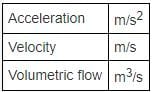RRB JE EEE (CBT I) Mock Test- 7 - Question 92

Chemical elements which belong to the group 18 of periodic table are called___________.

Detailed Solution for RRB JE EEE (CBT I) Mock Test- 7 - Question 92 Noble gases are the chemical elements which belong to group 18 of the periodic table.

The elements are- helium, neon, argon, krypton, xenon, and radon.

Having optimum valence electrons in their outer shell, they are the most stable elements found in environment.

RRB JE EEE (CBT I) Mock Test- 7 - Question 93

Statement: Refrigerators work on the principle of reverse heat engine.

Detailed Solution for RRB JE EEE (CBT I) Mock Test- 7 - Question 93 Reverse heat engine is a device that transfers energy from an object at a lower temperature to an object at a higher temperature by doing work on the system which is essentially reverse of the process that takes place inside a heat engine, in which energy flows from a higher to a lower temperature and work is generated as a result.

Refrigerators and Air conditioners are typical example of reverse heat engines.

Efficiency does not play any role in the simple working of any of these processes.

RRB JE EEE (CBT I) Mock Test- 7 - Question 94

Which of the following is the semi fluid substance upon with organelles of the animal cell are found?

Detailed Solution for RRB JE EEE (CBT I) Mock Test- 7 - Question 94 Cytoplasm is the semi fluid substance enclosed within the cell membrane of an animal cell.

Its constituents are- water (80 - 85%), proteins (10 - 15%), lipids (2 - 4%) and rest are polysaccharides and nucleic acids.

All the organelles like Ribosomes, Mitochondria, golgi apparatus etc. are found in this.

RRB JE EEE (CBT I) Mock Test- 7 - Question 95

Alexander Fleming was a Scottish physician & pharmacologist who is best known for his discovery of ____________.

Detailed Solution for RRB JE EEE (CBT I) Mock Test- 7 - Question 95 In 1928 Alexander Fleming discovered penicillin, and after successful decades of its vital use and importance received the Nobel Prize in Medicine in 1945.

Fleming's discovery of penicillin occurred in 1928, while he was investigating a common type of bacteria that causes boils and infections in patients with weakened immune systems.

RRB JE EEE (CBT I) Mock Test- 7 - Question 96

Bronchitis is an ailment related to which of the following internal organ of the Human body?

Detailed Solution for RRB JE EEE (CBT I) Mock Test- 7 - Question 96 Bronchitis occurs when bronchial tubes, which essentially carry air to the lungs in the human system, gets infected and swollen causing a massive build-up of mucus

Same viruses that causes cold or the flu also cause bronchitis.

RRB JE EEE (CBT I) Mock Test- 7 - Question 97

Which of the following process take place in a blast furnace in a metallic extraction industry?

Detailed Solution for RRB JE EEE (CBT I) Mock Test- 7 - Question 97 Smelting is a process to mix and melt metallic ores in order to control the pure metallic content according to specification in an industry.

Soldering is a process in which two or more metal items are joined together by melting and then flowing a filler metal into the joint - the filler metal having a relatively low melting point. Soldering is used to form a permanent connection between electronic components.

Forging is an after-extraction process where metal is given its desired shape.

RRB JE EEE (CBT I) Mock Test- 7 - Question 98

_____________ is the act or process of making soap.

Detailed Solution for RRB JE EEE (CBT I) Mock Test- 7 - Question 98 Saponification is an organic chemical reaction utilized to make soap.

Saponification is a process by which triglycerides are reacted with sodium or potassium hydroxide (lye) to produce glycerol and a fatty acid salt, called "soap."

There are 2 compounds involved in this process- Fat and an Alkali.

RRB JE EEE (CBT I) Mock Test- 7 - Question 99

Which of the following is a 2-terminal diode which stores electrical energy and used in everyday appliances?

Detailed Solution for RRB JE EEE (CBT I) Mock Test- 7 - Question 99 The capacitor is made of two closely placed conducting plates separated by a dielectric material.

The plates accumulate electric charge when connected to the power source. One plate accumulates the positive charge and the other plate accumulates a negative charge.

The capacity of a capacitor to store electrical energy is called Capacitance.

RRB JE EEE (CBT I) Mock Test- 7 - Question 100

Which of the following is responsible for the floating of the brain in the Human skull?

Detailed Solution for RRB JE EEE (CBT I) Mock Test- 7 - Question 100 In the human skull, the brain "float" in the cerebrospinal fluid. It is found within the skull and spine. This cushioning fluid is produced by the choroid plexus tissue, which is located within the brain, and flows through a series of cavities (ventricles) out of the brain and down along the spinal cord.

The cerebrospinal fluid is kept separate from the blood supply by the blood-brain barrier

## RRB JE Mock Test Series Electrical & Electronics Engineering

11 tests
 Use Code STAYHOME200 and get INR 200 additional OFF Use Coupon Code
Information about RRB JE EEE (CBT I) Mock Test- 7 Page
In this test you can find the Exam questions for RRB JE EEE (CBT I) Mock Test- 7 solved & explained in the simplest way possible. Besides giving Questions and answers for RRB JE EEE (CBT I) Mock Test- 7, EduRev gives you an ample number of Online tests for practice

11 tests